Electricity and Magnetism

Kirchoff's Current Law (conservation at nodes)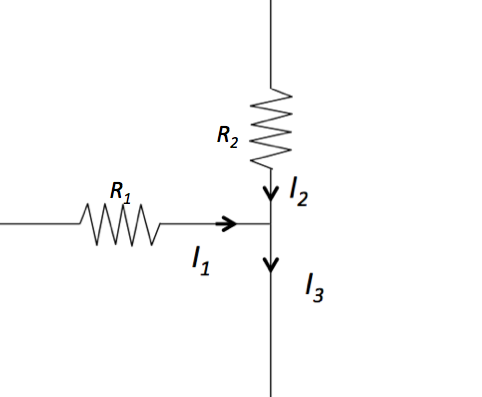The above figure shows part of a circuit. When the currents coming through $R_1 = 6 \Omega$ and $R_2 = 4 \Omega$ are $I_1 = 8 \text{ A}$ and $I_2 = 2 \text{ A},$ respectively, what is $I_3?$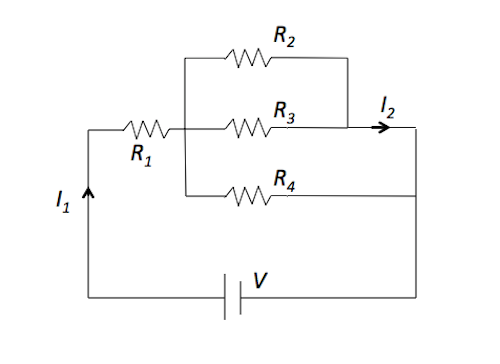In the above circuit, the resistance $R_1$ is $8 \Omega$ and the other resistances $R_2, R_3,$ and $R_4$ are $4 \Omega$ each. If $I_1 = 21 \text{ A},$ what is the current $I_2?$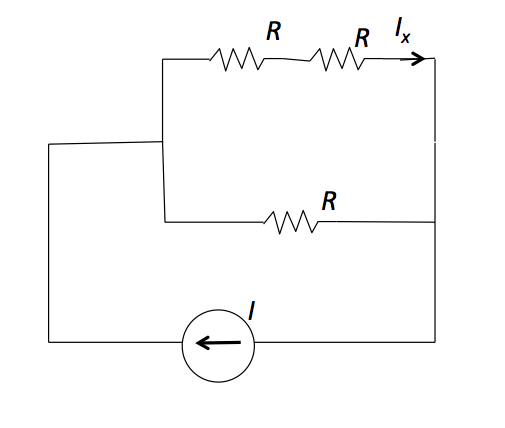In the above circuit, the current source flow is $I = 21 \text{ A}.$ When the resistances are given by $R = 3 \Omega,$ find the current $I_x.$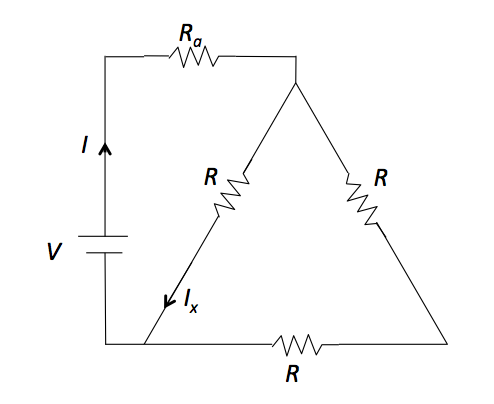In the above circuit, let the resistances $R_a = 7 \Omega$ and the resistances $R = 2 \Omega.$ The voltage of battery is unknown but the current is $I = 18 \text{ A}.$ Find the current $I_x$ in the above circuit.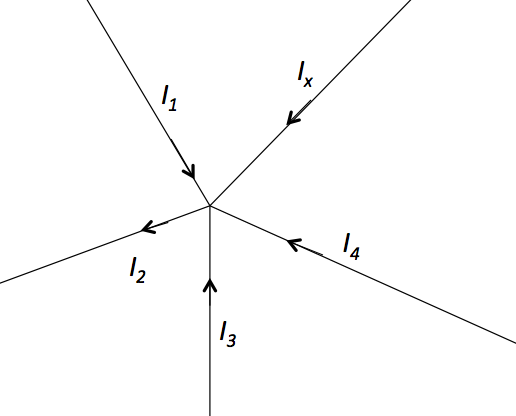In the above circuit, $I_1 = 4 \text{ A},$ $I_2 = 8 \text{ A},$ $I_3 = 3 \text{ A}$ and $I_4 = 3 \text{ A}.$ What is $I_x?$

×